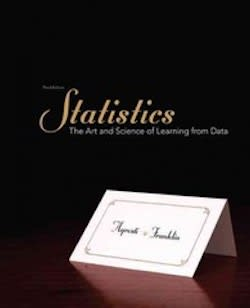Statistics Statistics: The Art and Science of Learning From Data# Statistics: The Art and Science of Learning From Data Agresti • 3rd Edition • 978-0321755940

Not the textbook you were looking for? Pick another one here.

## Ch. 2 - Exploring Data with Graphs and Numerical Summaries

Qualitative Data Quantitative Data Measures of Central Tendency Measures of Spread: Standard Deviation Measures of Spread: Interquartile Range Boxplots (The Five Number Summary)

## Ch. 6 - Probability Distributions

The Binomial Distribution The Binomial Formula The Binomial Table The Normal Distribution The Z-Score The Z-Table Probabilities from Scores Scores from Probability

## Ch. 7 - Sampling Distributions

Sampling Distribution for a Sample Mean Sampling Distribution for a Sample Proportion

## Ch. 8 - Statistical Inference: Confidence Intervals

Large Sample Confidence Interval for a Population Mean Small Sample Confidence Interval for a Population Mean Confidence Interval for a Population Proportion Sample Size

## Ch. 9 - Statistical Inference: Significance Tests About Hypotheses - Part 1 (Basics)

The Idea Behind Hypothesis Testing Type 1 & Type 2 Errors Null and Alternative Hypotheses Critical Values & Rejection Regions Large Sample Test Statistic: Mean Small Sample Test Statistic: Mean Test Statistic: Proportion